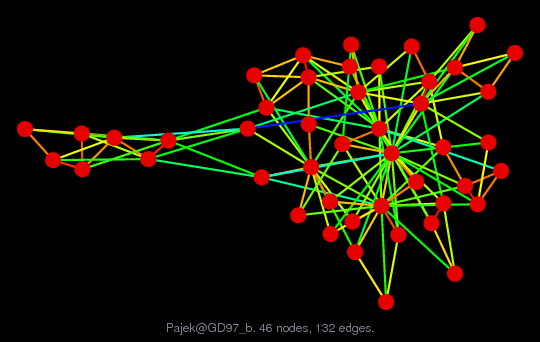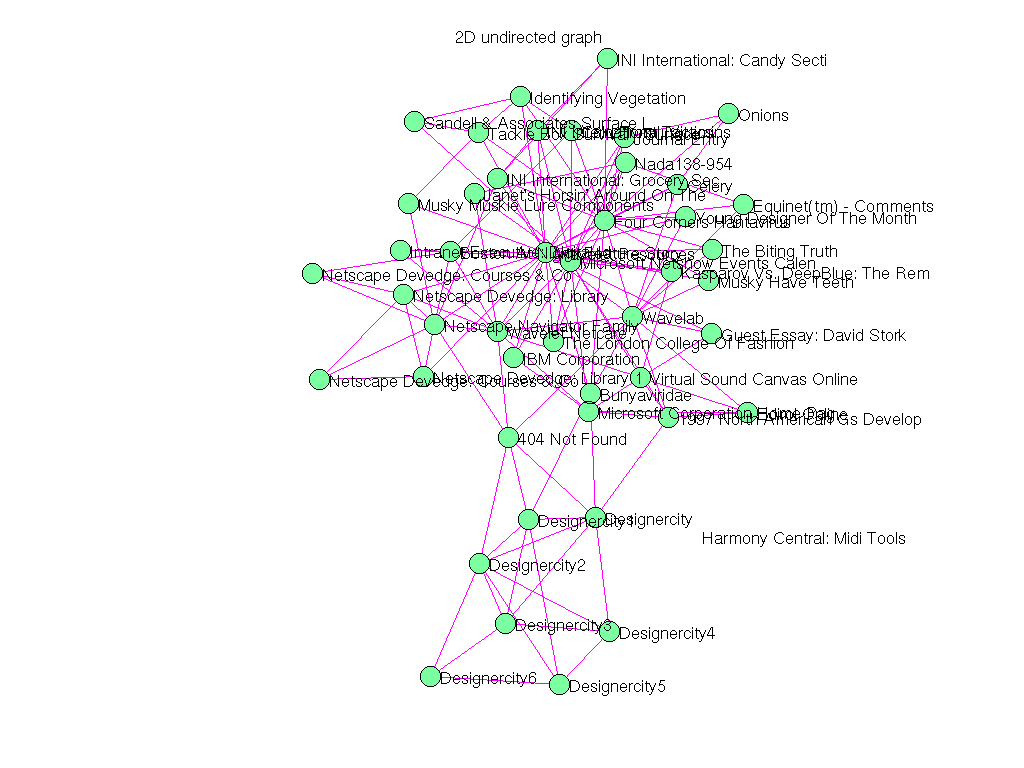Matrix: Pajek/GD97_b

Description: Pajek network: Graph Drawing contest 1997(undirected graph drawing)• Matrix group: Pajek
• download as a MATLAB mat-file, file size: 4 KB. Use UFget(1493) or UFget('Pajek/GD97_b') in MATLAB.

 Matrix properties number of rows 47 number of columns 47 nonzeros 264 # strongly connected comp. 2 explicit zero entries 0 nonzero pattern symmetry symmetric numeric value symmetry symmetric type real structure symmetric Cholesky candidate? no positive definite? no

 author Graph Drawing Contest editor V. Batagelj date 1997 kind undirected weighted graph 2D/3D problem? no

 Additional fields size and type nodename full 47-by-30 coord full 47-by-2

Notes:

```------------------------------------------------------------------------------
Pajek network converted to sparse adjacency matrix for inclusion in UF sparse
matrix collection, Tim Davis.  For Pajek datasets, See V. Batagelj & A. Mrvar,
------------------------------------------------------------------------------
Regarding conversion for UF sparse matrix collection: in the original data
every edge appears exactly twice, with the same edge weight.  It could be a
multigraph, but it looks more like a graph.  The duplicate edges are removed
in this version.  You can always add them back in yourself; just look at 2*A.
------------------------------------------------------------------------------
The original problem had 3D xyz coordinates, but all values of z were equal
to 0.5, and have been removed.  This graph has 2D coordinates.
```

 SVD-based statistics: norm(A) 2841.06 min(svd(A)) 0 cond(A) Inf rank(A) 44 null space dimension 3 full numerical rank? no singular value gap 8.48707e+09

 singular values (MAT file): click here SVD method used: s = svd (full (A)) status: ok